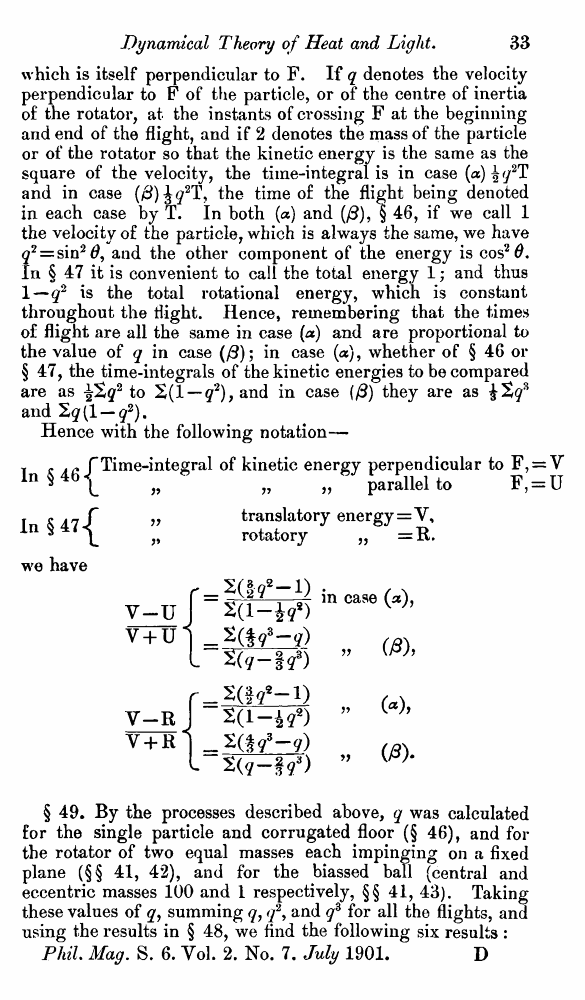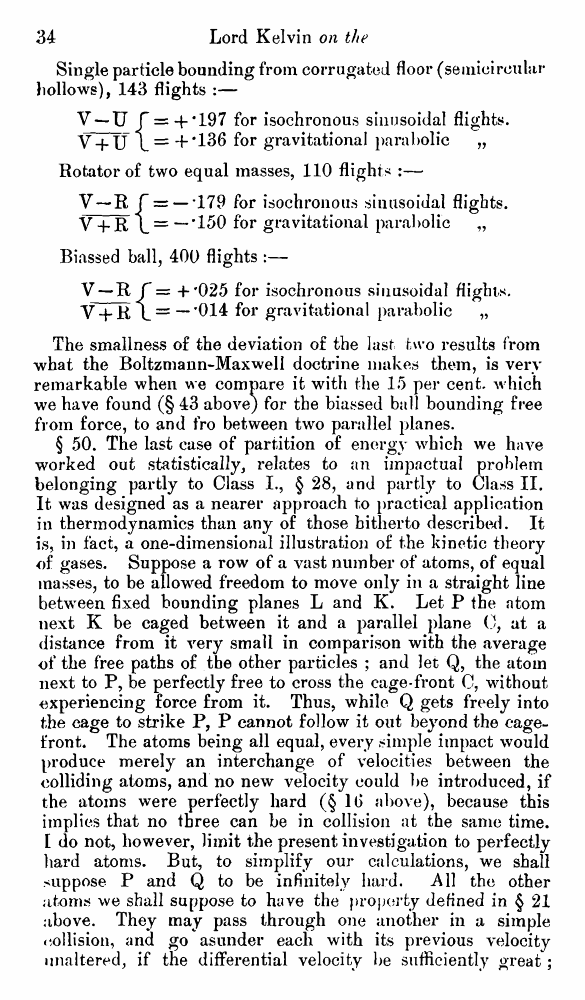# Lord Kelvin. Nineteenth-Century Clouds over the Dynamical Theory of Heat and Light. // Phil. Mag. S. 6. Vol. 2. No. 7. July 1901.

В начало   Другие форматы   <<<     Страница 33   >>>1  2  3  4  5  6  7  8  9  10  11  12  13  14  15  16  17  18  19  20  21  22  23  24  25  26  27  28  29  30  31  32  33 34  35  36  37  38  39  40 which is itself perpendicular to F. If q denotes the velocity perpendicular to F of the particle, or of the centre of inertia of the rotator, at the instants of crossing F at the beginning and end of the flight, and if 2 denotes the mass of the particle or of the rotator so that the kinetic energy is the same as the square of the velocity, the time-integral is in case (a) ^q2T and in case (#) the time of the flight being denoted in each case by T. In both (a) and (/3), § 46, if we call 1 the velocity of the particle, which is always the same, we have q2 = sin2#, and the other component of the energy is cos2#. In § 47 it is convenient to call the total energy 1; and thus 1—q2 is the total rotational energy, which is constant throughout the flight. Hence, remembering that the times of flight are all the same in case (a) and are proportional to the value of q in case (/3); in case (a), whether of § 46 or § 47, the time-integrals of the kinetic energies to be compared are as ^2g2 to 2(1 — q2), and in case (/3) they are as £2 above), because this implies that no three can be in collision at the same time. [ do not, however, limit the present investigation to perfectly hard atoms. But, to simplify our calculations, we shall suppose P and Q to be infinitely hard. All the other atoms we shall suppose to have the property defined in § 21 above. They may pass through one another in a simple collision, and go asunder each with its previous velocity unaltered, if the differential velocity be sufficiently great;